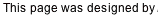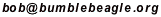## Solution Guide (without using the strings)

In this discussion, it will be useful to refer to the bulbs with single letters (see the list below). It will also be useful to refer to a cell in the square with a lowercase letter for a column, and a number for a row. Regions will be refered to by the letter of the hint bulb given in that region. Hint blubs are circled in these diagrams so you can keep 'em straight.

Text will refer to the diagram above it. The descriptions will start out fairly detailed, but become briefer as the solution progresses.

A stands for an aqua bulb (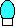).
B stands for a blue bulb (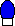).
G stands for a green bulb (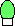).
O stands for an orange bulb (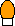).
P stands for a purple bulb (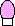).
R stands for a red bulb (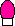).
Y stands for a yellow bulb (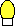).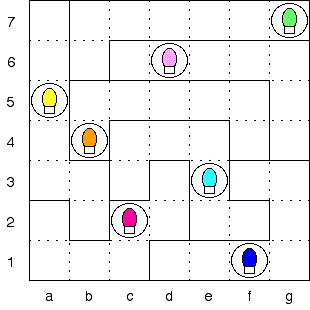So here's what we start with. As an example of the terminology, cell e3 contains an A, and cell d3 is part of region R.

As was noted in the puzzle instructions, g5=O, since this is the only place in row 5 that an O can go; the other empty cells in that row can't be O because they are part of a region that already contains an O (region O).

Similarly, f4=Y. This is the only cell in region O that can contain a Y.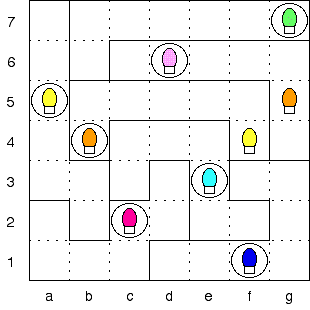Now a6=O; b6 can't be O because there's an O in the same column; and the remainder of the row is in region P, which already contains an O. So a6 is the only place left for an O in row 6.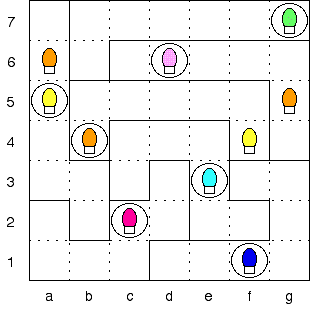Now consider a7 and b6. Whatever ends up in a7 cannot be anywhere else on row 7. But region G must have one bulb of that color, and the only place left for it will be at b6. So these cells must have the same color bulbs; a7=b6.

Similarly, whatever is at b6 will be eliminated from most of region P. The only spot left will be g4. So a7=b6=g4. We don't know what color bulb is at these cells, but it is useful to know that they must all be the same.

Looking at the lower left, we find a similar situation. Whatever ends up in a2 cannot be anywhere else in region Y except b3. So a2=b3. And for the same reason, a1=b2.

In row 4, a4 and g4 are the only cells can be A (the other empty cells are in region A). Let's suppose that g4=A. Then so are a7 and b6.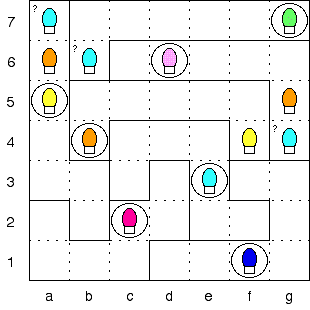With g4=A, d1=A (region) and c5=A (column). But then the only place for the last A would be at f2, and f2 is in a region that already has an A.

So g4 is not A, and thus a4=A.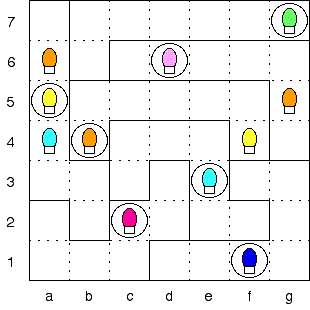a7 is either R or B. Suppose it's R; then so are b6 and g4.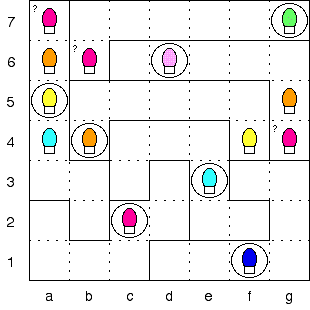With a7=R, f3=R (row). But then there is no place for R in row 1.

So a7 is not R, and thus a7=b6=g4=B.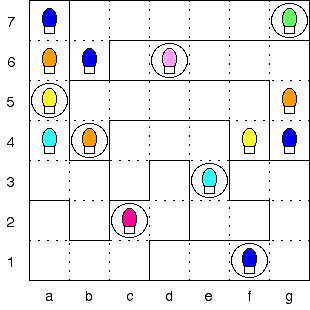a3=R (column).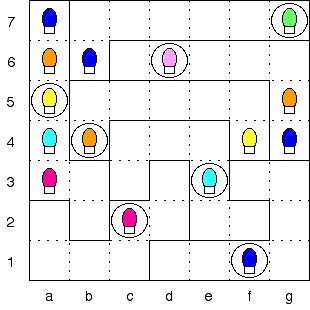a1 and a2 are P and G, in either order (column); Suppose a1=P and a2=G; then b2=P and b3=G.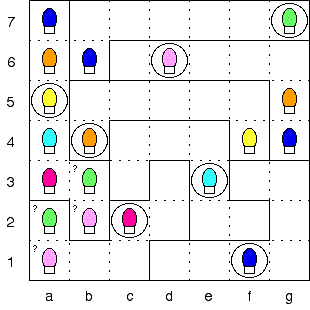With a1=P, g3=P (column). Then f3=O (only choice left for this cell). But then there is no bulb color that works in f2. So a1 is not P, and thus a1=b2=G and a2=b3=P.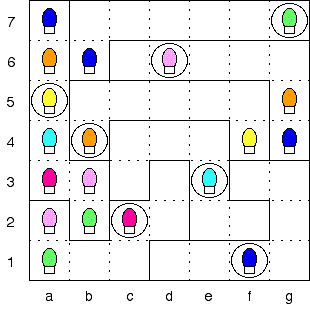g1=P (column), f3=G (region), g6=R (column), g2=A (column), g3=Y (column).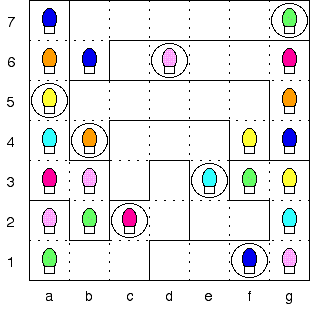At this point everything falls like dominoes. f6=A (only), f6=O (only), e6=Y (region), d7=Y (column), e5=B (column), c6=Y (row), e6=G (row), d6=B (row), d5=O (column), c5=B (row), d5=A (column), b5=R (row), f5=P (row), c5=G (row), d7=R (only), e7=O (region), d4=G (column), f7=R (column), e7=P (column), e4=R (column), c4=P (row), c7=A (only), c7=O (only), b7=Y (only), and (drum roll, please) b7=A (only).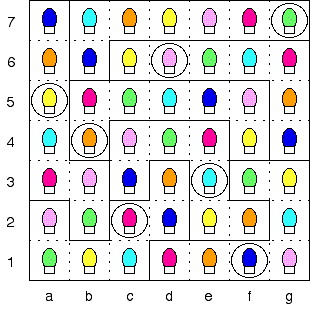Puzzle solved!

Back to Rooftop Lights Puzzle